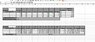# Convergent Divergent Nozzle

JRS192
Hi,
I hope that, given I've sourced what I can, you may be able to help? I'm Currently working on a lab report for my Aeroengines unit based on Convergent-Divergent nozzle (http://imgur.com/HQML2Au).

From the drawing, I have been provided D1, D2, D3, D4, T1, T2, T3, T4, P1, P2, P3, Velocity, Ambient Pressure and Ambient Temperature.

I need to calculate the following:
- Mass Air Flow rate through the system at each inlet valve.
- Velocity at each section (inlet, throat, exit) of the nozzle.
- Speed of Sound at each section (inlet, throat, exit) of the nozzle.
- Mach No. at each section (inlet, throat, exit) of the nozzle.

I don't have a real grasp of this subject, but from some text-books I've figured out the following, and mainly, I just want to ensure what I'm doing is correct.

•Mass Flow Rate
Density x Surface Area x Velocity Density = pRT, so I assume I'd take P1, and divide by (T1 x Universal Gas Constant)
Surface Area = (Pi x D12)/4
Velocity = Provided as part of the experiment.

Velocity
Continuity Equation = p1A1V1=p2A2V2 Now that I have p1 and A1 from the previous part, I should be able to rearrange to get V1? I'm assuming this would be the same for V3, V4?

Speed of Sound
I have this for a perfect gas: a = ROOT(yGcRT) So Root(specific heat ratio(?) x Newton's Constant x Gas Constant x Temperature?)
Not sure how to calculate each part of this

Mach No. M2 = V2/(specific heat ratio(?) x Newton's Constant x Gas Constant x Temperature?)

Any help at all would be appreciated, I've worked out what I can, as I don't want to flat-out ask for answers...

Thank you! :)

Mentor
I see a mass balance, but I don't see any momentum or energy balances. Have you heard of Bernoulli's equation?

Chet

JRS192
Hi Chet,

Thank you for responding - I've not been given anything other than what's above, can I calculate momentum or energy from those?

I've heard Bernoulli's in passing, but its not something we've covered; is this along the right lines? http://hyperphysics.phy-astr.gsu.edu/hbase/pber.html#bcal

I also have the attached presentation

#### Attachments

• Convergent Divergent Nozzles.pdf
725.6 KB · Views: 1,225
Last edited:
Mentor
You speak of "inlet valves." Do you really mean that gas is flowing into the nozzle through the side tubes, or are these just pipes at which the pressure and temperature are measured? Let's see your calculations so far.

Chet

JRS192
Hi Chet,

The temperatures are measured at those points.
I've not fully calculated everything, but this is my initial attempt

Thank you!

#### Attachments

• doc00552020150113165236.pdf
586.9 KB · Views: 226
•Capture.JPG
47.8 KB · Views: 580
Mentor
Hi Chet,

The temperatures are measured at those points.
I've not fully calculated everything, but this is my initial attempt

Thank you!
Well, you know that the gas densities are not supposed to be negative, right? So, what did you do wrong in applying the ideal gas law to get the densities? Hint: Those pressures are not absolute pressures... they are gage pressures.

This problem does not look like an exercise in how to apply conservation of momentum and conservation of energy to solve a problem for nozzle operation. It is designed to give you practice in applying the relevant equations to data in order to diagnose the operation of a nozzle.

Chet

JRS192
Well, you know that the gas densities are not supposed to be negative, right? So, what did you do wrong in applying the ideal gas law to get the densities? Hint: Those pressures are not absolute pressures... they are gage pressures.

This problem does not look like an exercise in how to apply conservation of momentum and conservation of energy to solve a problem for nozzle operation. It is designed to give you practice in applying the relevant equations to data in order to diagnose the operation of a nozzle.

Chet
Thought the negatives were a bit suspicious...thanks for that! I'm guessing I'd need to sub the Ambient pressure in instead?

Do the equations look ok?

Mentor
Thought the negatives were a bit suspicious...thanks for that! I'm guessing I'd need to sub the Ambient pressure in instead?

Do the equations look ok?
The absolute pressures equal 1 bar plus the gage pressures. I'm guessing that, when you do this, all the mass flow rates will come out the same.

The equations look OK because they are taken straight from the presentation. In applying the equations, please be careful to get the units right.

Chet